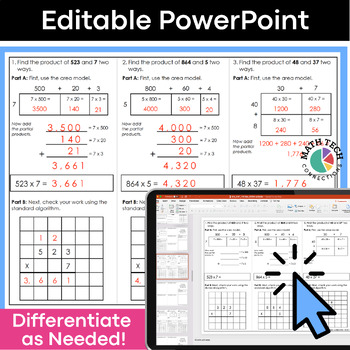# 5th Grade Place Value, Decimals, Multi-Digit Multiplication & Division5th
Subjects
Standards
Resource Type
Formats Included
• Zip
Pages

#### Also included in

1. These math tri-folds are perfect for you to use during your guided math groups, math assessments, or homework. Three tri-folds per standard give you plenty material to introduce, practice, and assess all 3rd grade math standards.This resource has been updated to include a Google Slides version with
\$25.00
\$37.50
Save \$12.50
2. This fifth grade math bundle includes 3 AMAZING MATH RESOURCES! Included: Math Tri-Folds, Math Sorts, and Math Morning WorkThis are MUST have resources for your 5th grade classroom. The resources included are flexible and may be used for morning work, whole group practice, guided math practice, inde
\$67.50
\$111.00
Save \$43.50
3. This 5th grade math bundle includes math tri-folds that are intended for use during small group instruction and Google Forms quizzes that can be used as pre and post math assessments. This 5th grade math practice bundle includes both PRINT and DIGITAL format. - The printable format includes 75 bookl
\$40.00
\$75.00
Save \$35.00

### Description

These 5th grade math tri-folds are perfect for you to use during your guided math groups, math assessments, or homework. Three tri-folds per standard give you plenty material to introduce, practice, and assess!

Topics Covered: Place Value, Powers of 10, Read/Write/Compare Decimals, Rounding Decimals, Multiplying Multi-Digit Numbers, Dividing Multi-Digit Numbers, Add/Subtract/Multiply/Divide Decimals

This resource has been updated to include a Google Slides version with simple text boxes, so you can use as Digital Math Worksheets.

These math booklets are organized by standard, so you will save time planning your small group lessons.

INCLUDED IN SET #2: Number & Operations in Base 10 (21 tri-folds)

These tri-folds cover the following standards:

5.NBT.1 : I can recognize that a digit in one place represents 10 times as much as it represents in the place to its right and 1/10 of what it represents in the place to its left.

5.NBT.1: I can recognize that a digit in one place represents 10 times as much as it represents in the place to its right and 1/10 of what it represents in the place to its left.

5.NBT.: I can explain patterns when multiplying a number by powers of 10. I can explain patterns in the placement of a decimal.

5.NBT.: I can read, write, and compare decimals to thousandths.

5.NBT.4: I can use place value understanding to round decimals to any place.

5.NBT.5: I can fluently multiply multi-digit whole numbers.

5.NBT.6: I can divide four-digit dividends by two-digit divisors. I can illustrate and explain the calculation by using equations, rectangular arrays, and/or area models.

5.NBT.7: I can add, subtract, multiply, and divide decimals to the hundredths place value. I can explain using concrete models or drawings.

* Editable Small Group Lesson Plan Template

* Level of Mastery Pages

WAYS TO USE:

* Introduce a specific standard whole group

* Use in small group to practice/reteach a specific standard

* Place in an independent math workshop center

* Use the 3rd tri-fold as an ASSESSMENT!

PURCHASE THE BUNDLE & SAVE!

5th Grade Math Tri-folds a total of 75 booklets!

PURCHASE SEPARATELY:

Set #1: Operations and Algebraic Thinking (9 tri-folds)

Set #2: Number & Operations in Base 10 (21 tri-folds)

Set #3: Number & Operations - Fractions (21 tri-folds)

Set #4 : Measurement & Data (12 tri-folds)

Set #5 : Geometry (12 tri-folds)

MORE MATH TRI-FOLDS

3rd Grade - Written Response Tri-Folds

4th Grade - Written Response Tri-Folds

5th Grade - Written Response Tri-Folds

Total Pages
Included
Teaching Duration
N/A
Report this Resource to TpT
Reported resources will be reviewed by our team. Report this resource to let us know if this resource violates TpT’s content guidelines.

### Standards

to see state-specific standards (only available in the US).
Add, subtract, multiply, and divide decimals to hundredths, using concrete models or drawings and strategies based on place value, properties of operations, and/or the relationship between addition and subtraction; relate the strategy to a written method and explain the reasoning used.
Find whole-number quotients of whole numbers with up to four-digit dividends and two-digit divisors, using strategies based on place value, the properties of operations, and/or the relationship between multiplication and division. Illustrate and explain the calculation by using equations, rectangular arrays, and/or area models.
Fluently multiply multi-digit whole numbers using the standard algorithm.
Use place value understanding to round decimals to any place.
Compare two decimals to thousandths based on meanings of the digits in each place, using >, =, and < symbols to record the results of comparisons.